# Introduction to R - exercises

Please follow the following exercises to get familiar with R. Parts of the exercises follow A (very) short introduction to R by Paul Torfs & Claudia Brauer, Hydrology and Quantitative Water Management Group Wageningen University, The Netherlands

Compute the difference between 2012 and the year you started at your university and divide this by the difference between 2012 and the year you were born. Multiply this with 100 to get the percentage of your life you have spent at this university. Use brackets if you need them.

(2012 - 2004)/(2012 - 1984) * 100

##  28.57


Find help for the log function.

?log


Repeat Task 1, but with several steps in between. You can give the variables any name you want, but the name has to start with a letter. Additionally, calculate the following:

#### a)

$\frac{5}{5+345}$

#### b)

$2 \cdot \sin (90°)$ Mind the conversion between degrees and radians!

#### c)

$\sqrt{16}+\sqrt{25}$

#### d)

$\frac{\frac{5}{5+345}+2 \cdot \sin (90°)}{\sqrt{16}+\sqrt{25}}$

atUni <- 2012 - 2004
alive <- 2012 - 1984

atUni/alive * 100

##  28.57


a <- 5/(5 + 345)
a

##  0.01429


b <- 2 * sin(90 * pi/180)
b

##  2


c <- sqrt(16) + sqrt(25)
c

##  9


(a + b)/c

##  0.2238


Compute the sum of 4, 5, 8 and 11 by first combining them into a vector and then using the function sum. What are mean and median of this series?

vec <- c(4, 5, 8, 11)
sum(vec)

##  28

mean(vec)

##  7

median(vec)

##  6.5


Plot 100 random numbers following a Gaussian distribution with mean 11 and standard deviation 42 as a series of connected points and add their the mean as a horizontal grey line. Additionally, plot these random numbers in a histogram (showing densities on the y-axis) and as box plot.

vec <- rnorm(100, 11, 42)
plot(vec, type = "b")
abline(h = mean(vec), col = "gray")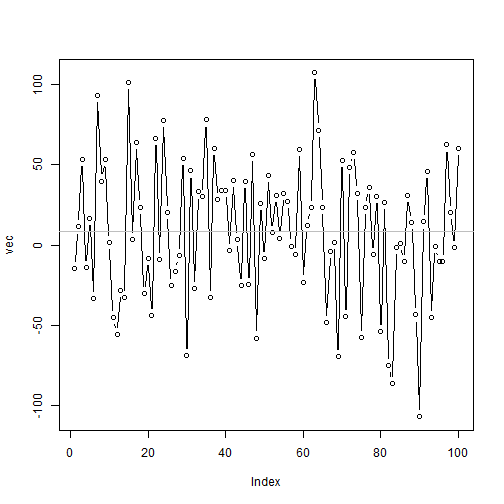hist(vec, n = 10, freq = F)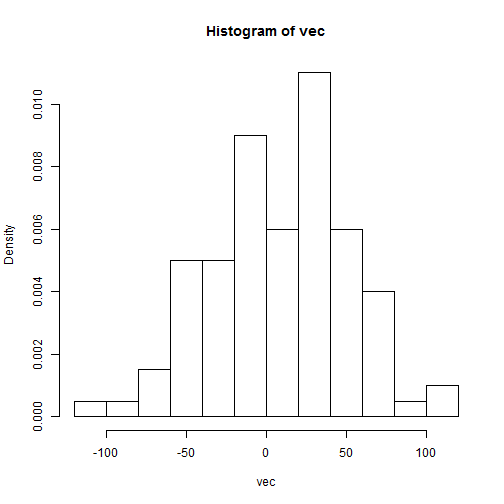boxplot(vec)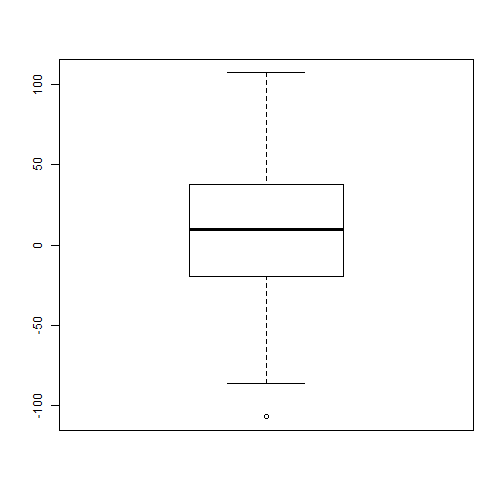Put the numbers 31 to 60 in a vector named p and in a matrix with 6 rows and 5 columns named q. Calculate the row- and column-wise sum of q.

p <- 31:60
q <- matrix(p, 6, 5)
q

##      [,1] [,2] [,3] [,4] [,5]
## [1,]   31   37   43   49   55
## [2,]   32   38   44   50   56
## [3,]   33   39   45   51   57
## [4,]   34   40   46   52   58
## [5,]   35   41   47   53   59
## [6,]   36   42   48   54   60

q <- matrix(p, 6, 5, byrow = T)
q

##      [,1] [,2] [,3] [,4] [,5]
## [1,]   31   32   33   34   35
## [2,]   36   37   38   39   40
## [3,]   41   42   43   44   45
## [4,]   46   47   48   49   50
## [5,]   51   52   53   54   55
## [6,]   56   57   58   59   60


apply(q, 1, sum)

##  165 190 215 240 265 290

apply(q, 2, sum)

##  261 267 273 279 285


Construct three random standard normal vectors of length 100. Call these vectors x1, x2 and x3. Make a data frame called t with three columns (called Va, Vb and Vc) containing respectively x1, x1+x2 and x1+x2+x3. Call the following functions for this data frame: plot(t) and cov(t). For each column call the function sd. Can you understand the results?

x1 <- rnorm(100)
x2 <- rnorm(100)
x3 <- rnorm(100)

t <- data.frame(Va = x1, Vb = x1 + x2, Vc = x1 + x2 + x3)
str(t)

## 'data.frame':    100 obs. of  3 variables:
##  $Va: num -1.683 -0.239 1.321 -0.417 0.33 ... ##$ Vb: num  -1.611 -2.613 0.558 -0.809 0.821 ...
##  $Vc: num -1.418 -2.427 0.814 -0.718 1.52 ...  plot(t)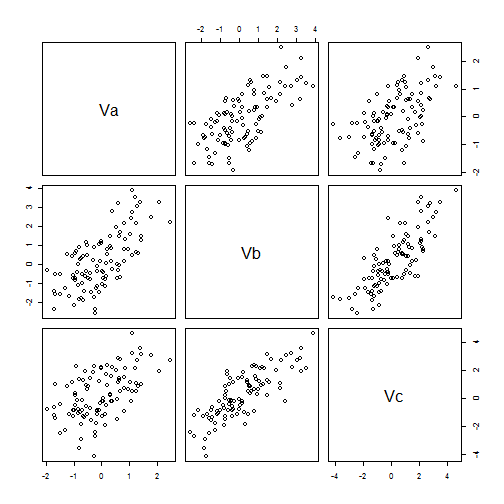cov(t)  ## Va Vb Vc ## Va 0.8454 0.9047 0.8312 ## Vb 0.9047 2.1204 1.9499 ## Vc 0.8312 1.9499 2.8123   apply(t, 2, sd)  ## Va Vb Vc ## 0.9194 1.4561 1.6770  lapply(t, sd)  ##$Va
##  0.9194
##
## $Vb ##  1.456 ## ##$Vc
##  1.677

sapply(t, sd)

##     Va     Vb     Vc
## 0.9194 1.4561 1.6770


Compute the mean of the square root of a vector of 100 random numbers. What happens?

smpl <- rnorm(100)
mean(sqrt(smpl))

## Warning: NaNs produced

##  NaN

mean(sqrt(abs(smpl)))

##  0.8278


sqrtSmpl <- sqrt(smpl)

## Warning: NaNs produced

sqrtSmpl

##       NaN    NaN 1.0123 1.4211 1.0484    NaN 0.7968 0.6534 1.2941 1.1250
##      NaN 0.6874 0.6137 0.4341    NaN    NaN    NaN    NaN 1.4721 0.5469
##   0.7369    NaN    NaN 0.7464    NaN 0.4092    NaN    NaN 0.5216 1.0074
##      NaN    NaN    NaN 0.2756 0.6043    NaN 1.4010 0.5813 0.4497    NaN
##   0.8242    NaN 1.0776    NaN 0.8118 1.0131 1.2734    NaN    NaN    NaN
##   1.2955 0.1381 0.7398    NaN    NaN 0.3028    NaN 0.9976 1.2344    NaN
##   1.0481 0.6593    NaN    NaN    NaN 0.8833 1.3619    NaN 0.7132    NaN
##      NaN 0.6165    NaN 0.2820 0.7753 1.3181    NaN 1.1700 0.1144    NaN
##   0.4681    NaN 0.3277 0.1482 1.1542    NaN 1.4893    NaN 0.9412    NaN
##      NaN    NaN    NaN    NaN 0.7589    NaN 0.9444    NaN    NaN    NaN

sqrtSmpl[is.nan(sqrtSmpl)] <- NA

mean(sqrtSmpl)

##  NA

mean(sqrtSmpl, na.rm = T)

##  0.8216


Make a vector from 1 to 100. Make a for-loop which runs through the whole vector. Multiply the elements which are smaller than 5 and larger than 90 with 10 and the other elements with 0.1.

x <- 1:100

for (i in 1:length(x)) {
if (x[i] < 5 | x[i] > 90)
x[i] <- x[i] * 5 else x[i] <- x[i] * 0.1
}
x

##      5.0  10.0  15.0  20.0   0.5   0.6   0.7   0.8   0.9   1.0   1.1
##     1.2   1.3   1.4   1.5   1.6   1.7   1.8   1.9   2.0   2.1   2.2
##     2.3   2.4   2.5   2.6   2.7   2.8   2.9   3.0   3.1   3.2   3.3
##     3.4   3.5   3.6   3.7   3.8   3.9   4.0   4.1   4.2   4.3   4.4
##     4.5   4.6   4.7   4.8   4.9   5.0   5.1   5.2   5.3   5.4   5.5
##     5.6   5.7   5.8   5.9   6.0   6.1   6.2   6.3   6.4   6.5   6.6
##     6.7   6.8   6.9   7.0   7.1   7.2   7.3   7.4   7.5   7.6   7.7
##     7.8   7.9   8.0   8.1   8.2   8.3   8.4   8.5   8.6   8.7   8.8
##     8.9   9.0 455.0 460.0 465.0 470.0 475.0 480.0 485.0 490.0 495.0
##  500.0


Write a function for the previous Task, so that you can feed it any vector you like (as argument). Use the standard R function length in the specification of the counter.

scaleFun <- function(x) {
for (i in 1:length(x)) {
if (x[i] < 5 | x[i] > 90)
x[i] <- x[i] * 5 else x[i] <- x[i] * 0.1
}

return(x)
}

scaleFun(1:100)

##      5.0  10.0  15.0  20.0   0.5   0.6   0.7   0.8   0.9   1.0   1.1
##     1.2   1.3   1.4   1.5   1.6   1.7   1.8   1.9   2.0   2.1   2.2
##     2.3   2.4   2.5   2.6   2.7   2.8   2.9   3.0   3.1   3.2   3.3
##     3.4   3.5   3.6   3.7   3.8   3.9   4.0   4.1   4.2   4.3   4.4
##     4.5   4.6   4.7   4.8   4.9   5.0   5.1   5.2   5.3   5.4   5.5
##     5.6   5.7   5.8   5.9   6.0   6.1   6.2   6.3   6.4   6.5   6.6
##     6.7   6.8   6.9   7.0   7.1   7.2   7.3   7.4   7.5   7.6   7.7
##     7.8   7.9   8.0   8.1   8.2   8.3   8.4   8.5   8.6   8.7   8.8
##     8.9   9.0 455.0 460.0 465.0 470.0 475.0 480.0 485.0 490.0 495.0
##  500.0

scaleFun(1:10)

##    5.0 10.0 15.0 20.0  0.5  0.6  0.7  0.8  0.9  1.0


Find a suitable marginal distribution for the Nile dataset:

data(Nile)
str(Nile)

##  Time-Series [1:100] from 1871 to 1970: 1120 1160 963 1210 1160 1160 813 1230 1370 1140 ...


plot(Nile)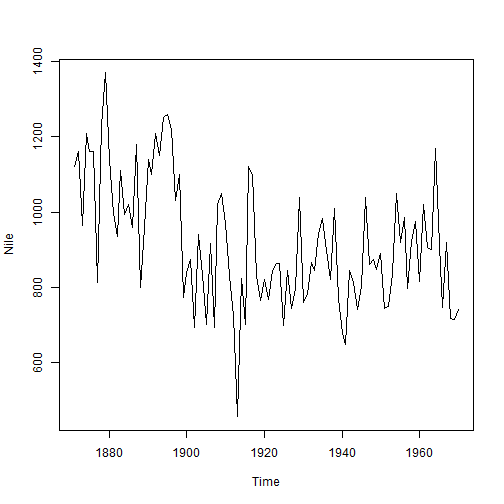hist(Nile, freq = F)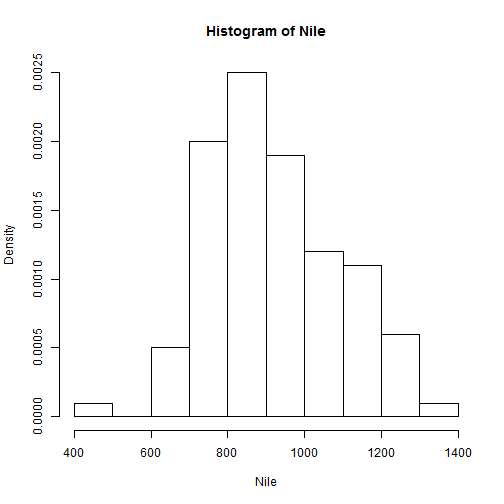optFunLogNorm <- function(param) {
-sum(log(dlnorm(as.vector(Nile), param, param)))
}

lnormFit <- optim(c(1, 1), optFunLogNorm)$par  ## Warning: NaNs produced  ## Warning: NaNs produced   dlnormFun <- function(x) dlnorm(x, lnormFit, lnormFit) # loglik sum(log(dlnormFun(as.vector(Nile)))) # -654  ##  -653.9   hist(Nile, freq = F, n = 20) curve(dlnormFun, add = T, col = "blue")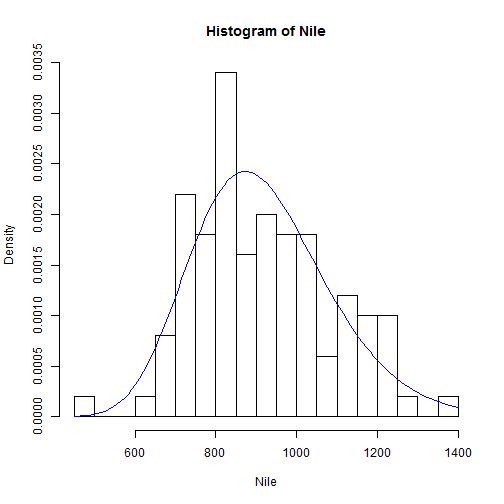## Task 12 Install all required packages for the course and take a look at their demos and data. install.packages("copula") install.packages("evd") demo(package="copula") demo(package="evd") data(package="copula") data(package="evd")  library("evd") data(uccle) str(uccle)  ## 'data.frame': 35 obs. of 4 variables: ##$ day : num  33.8 27.7 60 24 72.3 50.7 18.7 41.2 26.6 27.2 ...
##  $hour: num 14 12.8 12.9 11.9 20.6 29.1 6.2 21.1 11.2 18 ... ##$ tmin: num  6.5 8.5 5 8.4 13.2 11.9 3.8 13 11.1 13 ...
##  $min : num 2.5 1 0.5 0.9 1.5 4.4 1 3 3.3 2 ...  hist(uccle$hour)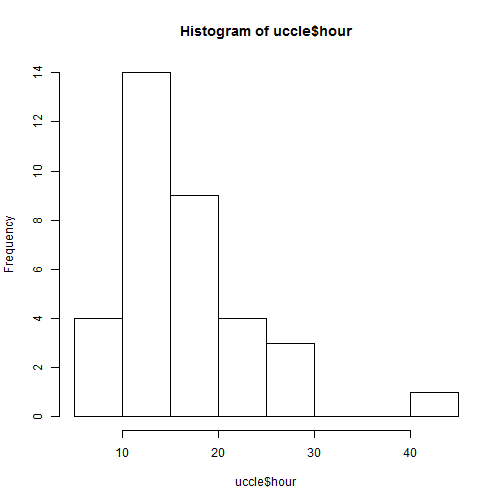Locate your favourite data set you might want to use throughout the course and load it into R. Make sure to save it as a clean .RData file. Mind your current working directory. Set up a new RStudio project for the upcoming week.

triples <- read.csv("simulatedTriples.csv")
save(triples, file = "myData.RData")

##### Optional extensions:

Install optional packages:

install.packages("sp")
install.packages("spacetime")
install.packages("VineCopula")
install.packages("rgl")
install.packages("spcopula", repos="http://R-Forge.R-project.org")


check the demo doing a subset of a multivariate return period analysis in the spcopula package:

library(spcopula)
demo("MRP")